﻿ How to read a boxplot - MathBootCamps

# How to read a boxplot

Boxplots are a way of summarizing data through visualizing the five number summary which consists of the minimum value, first quartile, median, third quartile, and maximum value of a data set. In the following lesson, we will look at how to use this information and the basic form of a boxplot to answer questions, therefore helping you understand how to read a boxplot.

## The basic form of a boxplot

If a data set has no outliers (unusual values in the data set), a boxplot will be made up of the following values.But, if there ARE outliers, then a boxplot will instead be made up of the following values.As you can see above, outliers (if there are any) will be shown by stars or points off the main plot. If there are no outliers, you simply won’t see those points. So, now that we have addressed that little technical detail, let’s look at an example to see what kinds of questions we can answer using a boxplot.

## Answering questions with a boxplot

The boxplot below shows the high temperatures in Anchorage, Alaska in May 2014*.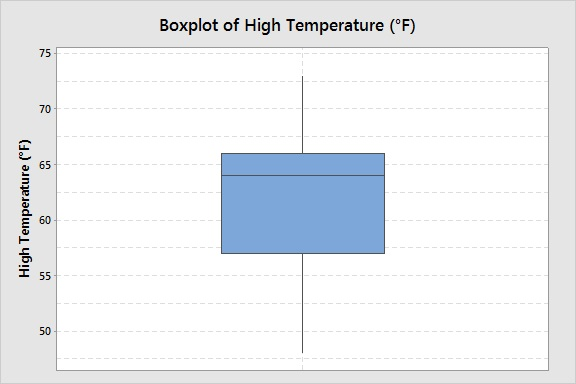Use this to answer the following questions.

(a) Are there any outliers in this data set?
(b) What was the lowest high temperature observed in May?
(c) Complete the sentence: “About 25% of days in May had high temperatures warmer than about ______ °F.”
(d) What was the median high temperature in May?
**(e) How many days in May did Anchorage see a high temperature of 65?
**(f) On what dates was the high temperature over 70°F?

Before we answer these, notice that this particular boxplot is vertical instead of horizontal. Depending on the software used, you may see either configuration. The basic form is the same for both.

### (a) Are there any outliers in this data set?

There are no stars or other points past the main line in the boxplot, so no, there are no outliers in this data set.

### (b) What was the lowest high temperature observed in May?

Since there are no outliers, the main line through the boxplot starts at the minimum value and ends at the maximum value. We are looking for the minimum value here.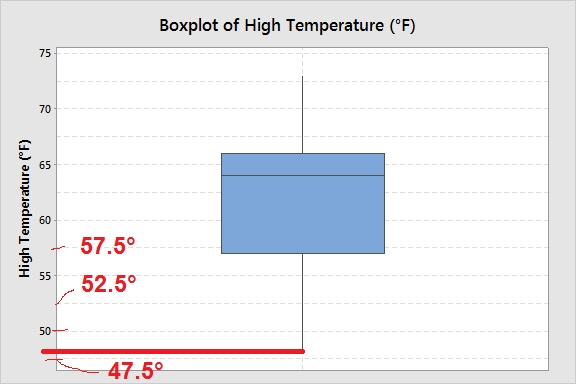First, you need to figure out the scale. Since every other line is labelled and it is counting by 5, the in between lines must represent 2.5°. The minimum looks just about 47.5°, so we will estimate it at 48° and as a final answer we can say “The lowest observed temperature in May was about 48°F.”

This is something you should be comfortable with. That is, we won’t always be able to give an exact answer from the graph depending on the scale. Without the actual data set, we will often have to estimate.

### (c) Complete the sentence: “About 25% of days in May had high temperatures warmer than about ______ °F.”

You may think that we need to be able to count values in the data set to answer this question, but actually we don’t! This is a question that can be answered using the fact that the boxplot shows the quartiles. When the data set is placed in order from smallest to largest, these divide the data set into quarters.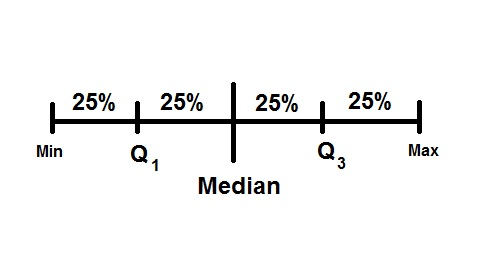From the picture:

• First quartile – Q1 – about 25% of a data set is smaller than the first quartile and about 75% is above.
• Third quartile – Q3 – about 75% of a data set is smaller than the third quartile and about 25% is above.

Now to actually answer the question! “Complete the sentence: “About 25% of days in May had high temperatures warmer than about ______ °F.” The third quartile is what we need to complete this sentence.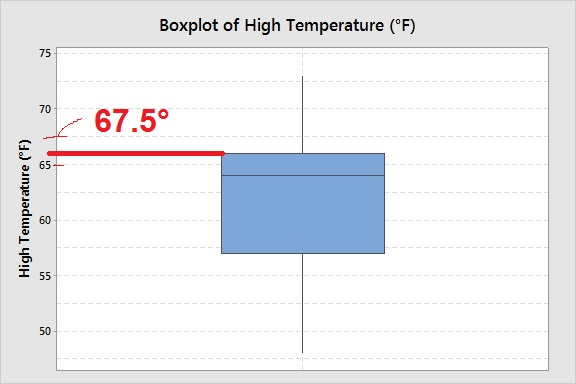It looks like the third quartile is about 66°. So we can write: About 25% of days in May had high temperatures warmer than about 66°F.

### (d) What was the median high temperature in May?

The median is shown by the line inside the box of the boxplot. This may not always be in the middle – it depends on the shape of the distribution among other things.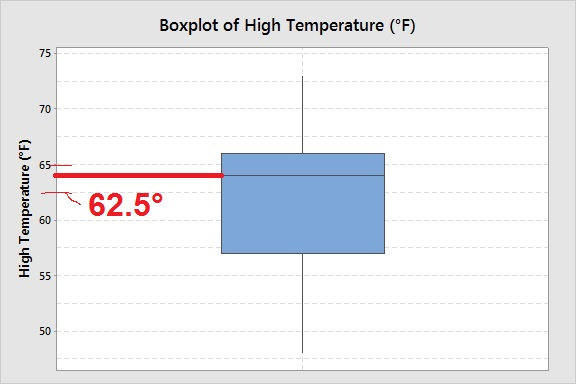The median for this data set is between 62.5°F and 65°F, and a bit closer to 65°F than not. I would estimate it at 64°F.

The median high temperature in May was about 64°F.

### (e) How many days in May did Anchorage see a high temperature of 65?

This question illustrates one weakness of a boxplot; a weakness that is shared with histograms. Information about individual data values isn’t shown. There is no way to answer this question with a boxplot. We would need to see a dotplot or a stemplot (or the data set itself) to be able to answer this question.

### (f) On what dates was the high temperature over 70°F?

Another question where it would be interesting to know the answer! Unfortunately, this is another case where some information is “lost” when making a boxplot. There is no way to tell which temperatures are from which dates. To see that, we would need to use a timeplot or simply a table.

## Conclusion

These last two questions show you that some plots, like boxplots and histograms, are designed to give you a big picture idea of a data set. Through this though, you lose some information about individual values. When making a plot of your own data set, you must consider whether this is important or not and select your plot accordingly.

*Source for this data: Weather Underground

**If you skipped down here, maybe you were suspicious of questions (e) and (f). You are right to be! These can’t be answered by a boxplot alone. The details are given in the answers.